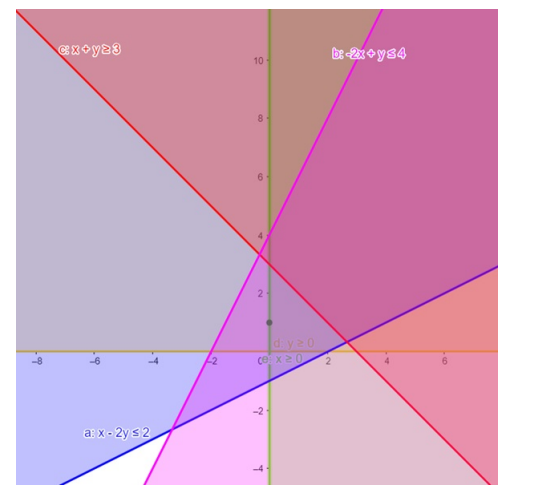# Solve the given inequalities

Question:

Solve the given inequalities $x-2 y \leq 2, x+y \geq 3,-2 x+y \leq 4, x \geq 0, y \geq 0 g r a p h i c a l l y$ in two - dimensional plane.

Solution:

The graphical representation of $x-2 y \leq 2, x+y \geq 3, y \geq 0$

$-2 x+y \leq 4, x \geq 0$ is given by common region in the figure below.

$x-2 y \leq 2 \ldots \ldots$ (1)

$x+y \geq 3 \ldots \ldots$ (2)

$x \geq 0 \ldots \ldots$ (3)

$y \geq 0 \ldots \ldots$ (4)

$-2 x+y \leq 4 \ldots \ldots \ldots$ (5)

Inequality (1) represents the region above line $x-2 y=2$ (including the line $x-2 y=2$ ).

Inequality (2) represents the region above line $x+y=3$ (including the line $x+y=3$ ).

Inequality (3) represents the region in front of line $x=0$ (including the line $x=0$ ).

Inequality (4) represents the region above line $y=0$ (including the line $y=0$ ).

Inequality (5) represents the region below line $-2 x+y=4$ (including the line $-2 x+y=4$ ).

Therefore,every point in the common shaded region including the points on the respective lines represents the solution for the given inequalities.

This can be represented as follows,